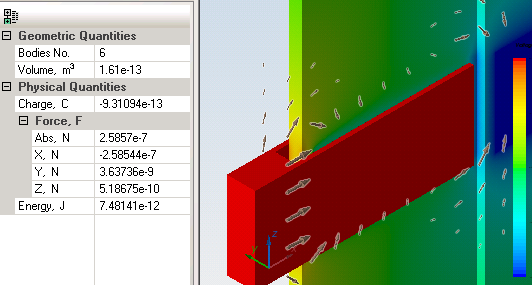Main >> Applications >> Sample problems

# Comb drive

microelectromechanical device simulation, comb drive electrostatic force, MEMS device simulation

Comb-drive is a MEMS device that utilize electrostatic forces acting between two electrically conductive combs connected to the voltage source.

Problem Type electrostatics.

Geometry 3D extrusion.

Comb thickness Lz = 40 um.
Gap between fingers d = 2.5 um.

Given
Relative permittivity of air ε = 1,
Relative permittivity of comb ε = 10,
Potential difference ΔU = 40 V

Find the attraction force per tooth width and compare with 2D analytical solution:*
F = 0.5 · εε0 · n·Lz · U² / d,
where n is number of air gaps.

Solution
The simulation model includes only one pair of teeth (two air gaps, n=2). The Dn=0 boundary condition is applied on the plane of symmetry.

Results
Analytical solution (force per one pair of electrodes, n=2):
F = 0.5 · 1 · 8.854e-12 · 2·40e-6 · 40² / 2.5e-6 = 2.27e-7 N.

 Force F Analytical solution 227 nN 2D plane simulation 229 nN 3D extrusion simulation 259 nN•